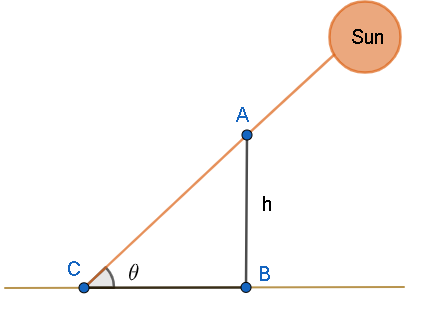Courses
Courses for Kids
Free study material
Offline Centres
MoreLast updated date: 06th Dec 2023
Total views: 282.3k
Views today: 4.82k

# Shadow of a vertical pillar is equal to the height of the pillar then the angle of elevation of sun will be:(a) $90{}^\circ$(b) $60{}^\circ$(c) $45{}^\circ$(d) $30{}^\circ$Verified
282.3k+ views
Hint: To find the angle of elevation of the sun, we have to draw a figure with the given data. We will then obtain a triangle. We have to use the formula $\tan \theta =\dfrac{\text{Opposite side}}{\text{Adjacent side}}$ to find the value of $\theta$ , that is, the angle of elevation of sun. According to the given condition, opposite and adjacent sides will be equal.

We are given that the shadow of a vertical pillar is equal to the height of the pillar. Let us draw a figure of this scenario.From the figure, we can see that when the sun rays fall on the top of the pillar of height AB, a shadow of length BC is created. From the given condition, we can write
$\text{AB}=\text{BC}$
Let us assume the height of the pole to be h.
$\Rightarrow \text{AB}=\text{BC}=h...\left( i \right)$
Now, we have to find the angle of elevation of the sun, that is, $\theta$ .
We know that $\tan \theta =\dfrac{\text{Opposite side}}{\text{Adjacent side}}$ .
Here, the opposite side is AB and the adjacent side is BC.
$\Rightarrow \tan \theta =\dfrac{\text{AB}}{\text{BC}}$
Let us substitute the value of AB and BC from (i) in the above equation.
\begin{align} & \Rightarrow \tan \theta =\dfrac{h}{h} \\ & \Rightarrow \tan \theta =1 \\ \end{align}
We know that $\tan 45{}^\circ =1$ . Let us substitute this value in the above equation.
\begin{align} & \Rightarrow \tan \theta =\tan 45{}^\circ \\ & \Rightarrow \theta =45{}^\circ \\ \end{align}
Therefore, the angle of elevation of the sun is $45{}^\circ$ .

So, the correct answer is “Option c”.

Note: Students must understand the question clearly and then draw a figure with the given data. Students must know to find the trigonometric angles using a triangle. We can find $\sin \theta$ by taking the ratio of the opposite side to the hypotenuse. Similarly, we can find $\cos \theta$ by taking the ratio of the adjacent side to the hypotenuse. Students must know the sides of the triangle. Also, they must note that we can apply these trigonometric formulas only when the given triangle is a right-angled one.